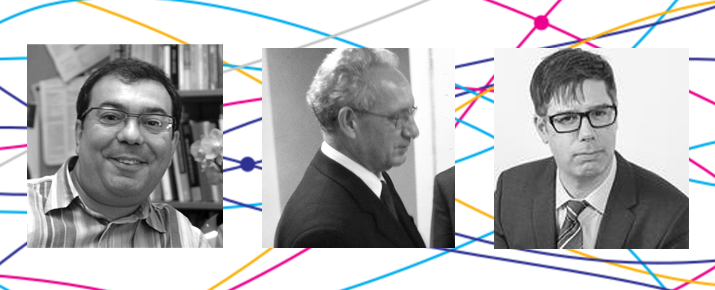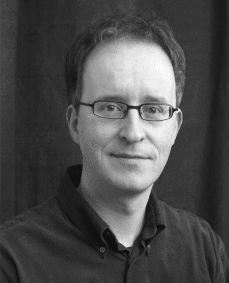# Shoenfield Logic Book and Article Prize Winners Announced

The Association for Symbolic Logic has awarded its 2022 Shoenfield Logic Book and Article Prizes.

The Shoenfield Prizes are “awarded for outstanding expository writing in the field of logic” and were established honor the late Joseph R. Shoenfield, a influential logician who died in 2000.

The Shoenfield Book Prize was awarded to Paolo Mancosu (University of California, Berkeley), Sergio Galvan (Catholic University of the Sacred Heart), and Richard Zach (Calgary) for their book, An Introduction to Proof Theory—Normalization, Cut-Elimination, and Consistency Proofs (Oxford University Press, 2021).Paolo Mancosu, Sergio Galvan, and Richard Zach

Here’s a summary of their book:

Proof theory is a central area of mathematical logic of special interest to philosophy. It has its roots in the foundational debate of the 1920s, in particular, in Hilbert’s program in the philosophy of mathematics, which called for a formalization of mathematics, as well as for a proof, using philosophically unproblematic, “finitary” means, that these systems are free from contradiction. Structural proof theory investigates the structure and properties of proofs in different formal deductive systems, including axiomatic derivations, natural deduction, and the sequent calculus. Central results in structural proof theory are the normalization theorem for natural deduction, proved here for both intuitionistic and classical logic, and the cut-elimination theorem for the sequent calculus. In formal systems of number theory formulated in the sequent calculus, the induction rule plays a central role. It can be eliminated from proofs of sequents of a certain elementary form: every proof of an atomic sequent can be transformed into a “simple” proof. This is Hilbert’s central idea for giving finitary consistency proofs. The proof requires a measure of proof complexity called an ordinal notation. The branch of proof theory dealing with mathematical systems such as arithmetic thus has come to be called ordinal proof theory. The theory of ordinal notations is developed here in purely combinatorial terms, and the consistency proof for arithmetic presented in detail.

The Shoenfield Article Prize was awarded to Vasco Brattka (Bundeswehr University Munich) for his article, “A Galois Connection between Turing Jumps and Limits”, published in Logical Methods in Computer Science in 2018.Vasco Brattka

Here’s the abstract of his article:

Limit computable functions can be characterized by Turing jumps on the input side or limits on the output side. As a monad of this pair of adjoint operations we obtain a problem that characterizes the low functions and dually to this another problem that characterizes the functions that are computable relative to the halting problem. Correspondingly, these two classes are the largest classes of functions that can be pre or post composed to limit computable functions without leaving the class of limit computable functions. We transfer these observations to the lattice of represented spaces where it leads to a formal Galois connection. We also formulate a version of this result for computable metric spaces. Limit computability and computability relative to the halting problem are notions that coincide for points and sequences, but even restricted to continuous functions the former class is strictly larger than the latter. On computable metric spaces we can characterize the functions that are computable relative to the halting problem as those functions that are limit computable with a modulus of continuity that is computable relative to the halting problem. As a consequence of this result we obtain, for instance, that Lipschitz continuous functions that are limit computable are automatically computable relative to the halting problem. We also discuss 1-generic points as the canonical points of continuity of limit computable functions, and we prove that restricted to these points limit computable functions are computable relative to the halting problem. Finally, we demonstrate how these results can be applied in computable analysis.

The Shoenfield prizes are awarded every three years. Any new book published during the nine years prior to the award year is eligible for the book prize; any article published during the six years prior to the award year is eligible for the article prize. You can see a list of previous prize winners here.Subscribe
Notify of